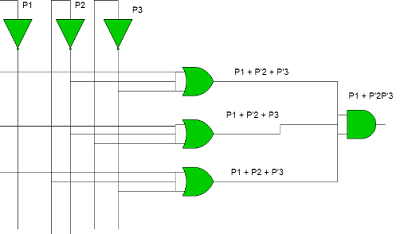# UGC NET COMPUTER SCIENCE SOLVED PAPERS 2017-19 - UGC NET Computer Science November 2017 Paper 2

>>>>>>>>UGC NET Computer Science November 2017 Paper 2

• A

K - Mean method• B

Self Organizing feature map method• C

K - nearest neighbor method• D

Agglomerative method• Option : C
• Explanation :
k-means clustering is a method of vector quantization, originally from signal processing, that is popular for cluster analysis in data mining.
Self-Organizing Map Self Organizing Map (SOM) provides a data visualization technique. SOM also represents clustering concept by grouping similar data together.
k-nearest neighbors algorithm (k-NN) is a non-parametric method used for classification and regression.
Agglomerative hierarchical clustering is a bottom-up clustering method where clusters have sub-clusters, which in turn have sub-clusters, etc.
Option (C) is correct.

• A

Zigbee• B

Bluetooth• C

Wi-Fi• D

GSM/CDMA• Option : B
• Explanation :
Internet of things(IoT) uses short range communication technology - Bluetooth. It’s an ultra low energy wireless technology for IOT devices which consumes the smallest amount of power in comparison to the already existing Bluetooth networking standards. So, option (B) is correct.

• A

Infinite• B

5• C

20• D

50• Option : C
• Explanation :
According to Amdahl's law speed up for infinite number of process:
S = 1 / (1-P)
where p is parallel part of program Given, sequential part of program is 5%. So parallel part of the program (P)
= 1 - sequential part
= 1 - 0.05 (or 5%)
= 0.95 (or 95%)
Now S = 1 / (1-P)
ie S = 1 / (1-0.95)
S = 1 / 0.05
S = 20
So, option (C) is correct.

• A

(1)• B

(2)• C

(3)• D

(4)• Option : B
• Explanation :
ince logic says that there exist some x for which H(x) is true, So the negation for this will be for all x H(x) is not true. We can write it:
¬∃xH(x) =∀x¬H(x)
So, option (B) is correct.

The value of F is:

• A

P1+P'2P3• B

P1+P'2P'3• C

P1+P2P'3• D

P'1+P2P3• Option : B
• Explanation :
We know that (A+B)(A+C) = A + BC ON the basis of Given question OR gates will produce output: (P1+P'2+P'3) (P1+P'2+P3) (P1+P2+P3) AND gate will take all three input produced by OR gate and generate (P1 + P'2P'3) as output.So, option (B) is correct.